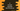# C program to convert seconds into hour/minute/seconds### C program to convert seconds into hour, minute and seconds :

In this tutorial, we will learn how to convert a seconds value to hours,minutes, and seconds. User will enter the second value, and our program will divide it into hours, minutes, and seconds. For example, 3600 means 1 hour,0 minute, and 0 seconds. Let’s take a look at the program :

### C program :

``````#include<stdio.h>

int main(){
//1
int inputSecond;

//2
int hours,minutes,seconds;
int remainingSeconds;

//3
int secondsInHour = 60 * 60;
int secondsInMinute = 60;

//4
printf("Enter seconds : ");
scanf("%d",&inputSecond);

//5
hours = (inputSecond/secondsInHour);

//6
remainingSeconds = inputSecond - (hours * secondsInHour);
minutes = remainingSeconds/secondsInMinute;

//7
remainingSeconds = remainingSeconds - (minutes*secondsInMinute);
seconds = remainingSeconds;

//8
printf("%d hour, %d minutes and %d seconds",hours,minutes,seconds);
}``````

### Explanation :

The commented numbers in the above program denote the step number below:

1. Create one integer variable to store the user-input seconds.

2. Create three integer variables hours,minutes, and seconds to store the final hour, minutes, and seconds value after splitting the user input value. Integer remainingSeconds is used to temporarily hold the remaining seconds value below.

3. secondsInHour denote the total number of seconds in one hour i.e. 3600 or 60 * 60. secondsInMinute denote the total seconds in one minute.

4. Ask the user to enter total seconds. Read and store it in variable inputSecond.

5. Find the hours by dividing the user input seconds by total seconds in one hour.

6. Calculate the remaining seconds by subtracting the total seconds in the calculated hour from the total user-given seconds. Using these remaining seconds, calculate the minutes by dividing the seconds by 60.

7. Again, calculate the current remaining seconds. These remaining seconds are the required value for seconds.

8. Print out all hours, minutes, and seconds values.

### Sample Output :

``````Enter seconds : 3600
1 hour, 0 minutes and 0 seconds

Enter seconds : 2345
0 hour, 39 minutes and 5 seconds

Enter seconds : 60
0 hour, 1 minutes and 0 seconds
``````

You might also like :